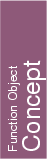## Kernel::ConstructBisector_3

A model for this must provide:

Kernel::Plane_3
 fo.operator() ( Kernel::Point_3 p, Kernel::Point_3 q)
constructs the bisector plane of p and q. The bisector is oriented in such a way that p lies on its positive side.
Precondition: p and q are not equal.

Kernel::Plane_3
 fo.operator() ( Kernel::Plane_3 h1, Kernel::Plane_3 h2)
constructs the bisector of the two planes h1 and h2. In the general case, the bisector has a normal vector which has the same direction as the sum of the normalized normal vectors of the two planes, and passes through the intersection of h1 and h2. If h1 and h2 are parallel, then the bisector is defined as the plane which has the same oriented normal vector as l1, and which is at the same distance from h1 and h2. This function requires that Kernel::RT supports the sqrt() operation.